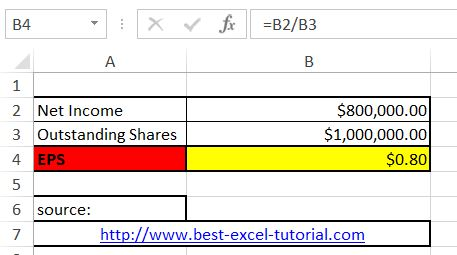# Earnings Per Share Calculator in Excel

Earnings per share (EPS) is a financial metric that measures a company’s profitability. In this lesson, you will learn how to calculate EPS in Excel.

Let’s build the earnings per share calculator in Excel. To calculate EPS in Excel first you need some data. You need: Net Income, Outstanding Shares and Preferred Dividends.

## How to calculate EPS?

The formula to calculate EPS is as follows:

Earnings Per Share = Net Income / Outstanding Shares

Copy and paste this eps formula into cell B4: =B2/B3

##How to calculate weighted EPS?

Weighted EPS accounts for situations where the number of outstanding shares changes during the period. This can occur due to stock splits, buybacks, or issuances. To calculate Weighted EPS, you need to adjust Net Income to account for Preferred Dividends (if any) and use the weighted average of outstanding shares.

The formula to calculate weighted EPS is as follows:

Weighted Earnings Per Share = (Net Income – Preferred Dividends) / Outstanding Shares

Copy and paste this roi formula in cell B5: =(B2-B3)/B4## Impact of Stock Splits

When a company undergoes a stock split, it affects the number of outstanding shares. To analyze the impact of a stock split on EPS, you’ll need to adjust the Outstanding Shares accordingly.

If a stock split involves a 2-for-1 split (where each shareholder receives two shares for every one held), you can use this formula:

= Outstanding Shares After Split = Outstanding Shares Before Split * Split Factor

= B3 * 2

Here, B3 represents Outstanding Shares Before Split, and the “2” in the formula represents the split factor for a 2-for-1 split.

Once you have the adjusted Outstanding Shares, you can recalculate EPS using the standard formula:

= Net Income / Outstanding Shares After Split

Note: The formula assumes that the net income and outstanding shares figures are up-to-date and accurate. It’s important to obtain this information from reliable sources, such as the company’s financial statements or financial data providers. Also, keep in mind that EPS is just one financial metric, and it’s important to consider a range of other factors when evaluating a company’s financial performance and potential for investment.